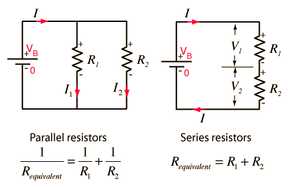297 Pages

## Problem

Consider three resistors with different resistivity,and. The formula for calculating the voltage across a resistor is.

Part 1: Determine the equivalent resistance when the three resistors are connected in series.

Part 2: Determine the equivalent resistance when the three resistors are connected in parallel.## Solution

Part 1: Resistors connected in series (same current, different voltage)

Letrepresent the voltage of the battery that follows Ohm's rule. Hererepresents the equivalent resistance of the resistors. When two resistors are connected in series, the current going through each resistor is the same, and the voltage drop across each resistor is proportional to their respective resistances. By Kirchoff's loop rule,Since,.

Therefore,In general, givenresistors added in seriesPart 2: Resistors connected in parallel (same voltage, different current)

When two resistors are connected in parallel, the voltage going through each resistor is the same, and the current the enters into the parallel branch is distributed across each resistor inversely-proportional to each resistor. By Kirchoff's junction rule,Since,.

Therefore,.

In general, givenresistors added in parallel.
Community content is available under CC-BY-SA unless otherwise noted.